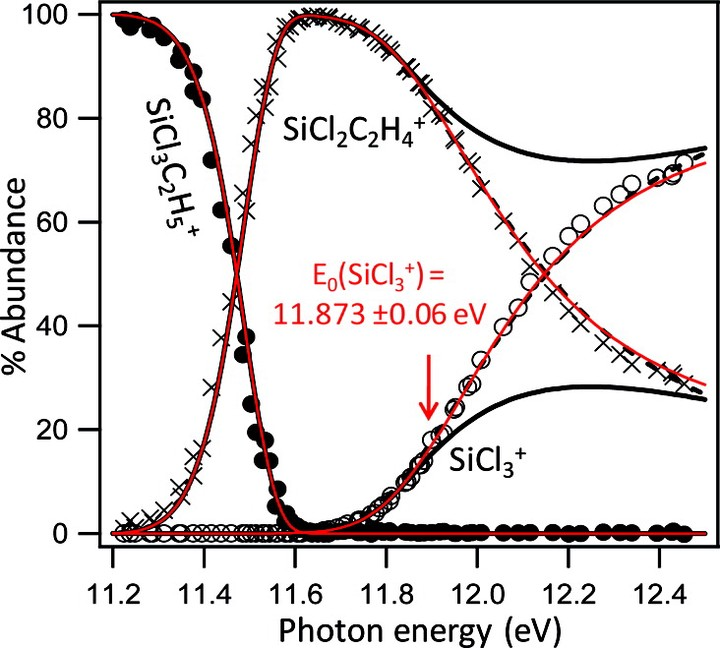# Experimental Thermochemistry of SiCl3R (R = Cl, H, CH3, C2H5, C2H3, CH2Cl, SiCl3), SiCl3+, and SiCl3•### Abstract

The 0 K onsets (E0) of a series of trichlorosilane derivatives SiCl3R ? SiCl3++ R? (R = Cl, H, CH3, C2H5, C2H3, CH2Cl, SiCl3) are measured by threshold photoelectron?photoion coincidence spectroscopy. The well-known heat of formation of SiCl4 is used as an anchor to determine the heat of formation of SiCl3+, which is, in turn, used as an anchor to determine the heats of formation of the other alkyltrichlorosilanes investigated. A series of isodesmic reactions at the G3 and CBS-QB3 levels are shown to accurately reproduce the experimental heats of formation, and this scheme is used to calculate the heat of formation of Si2Cl6, from which the measured E0 determines the SiCl3? heat of formation. The measured values then determine the IE of SiCl3? along with the Si?R bond dissociation enthalpies of the six neutral species investigated. The experimental heats of formation are also used in a series of isodesmic reaction calculations to determine the heats of formation of SiH3R (R = H, CH3, C2H5, C2H3, CH2Cl, SiCl3).

Publication
J. Phys. Chem. A